# Cuboctahedron

﻿
Cuboctahedron
Cuboctahedron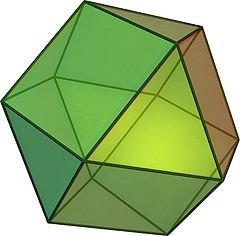Type Archimedean solid
Uniform polyhedron
Elements F = 14, E = 24, V = 12 (χ = 2)
Faces by sides 8{3}+6{4}
Schläfli symbol t1{4,3}
t0,2{3,3}
Wythoff symbol 2 | 3 4
3 3 | 2
Coxeter-DynkinSymmetry Oh, [4,3], (*432)
Td, [3,3], (*332)
Dihedral Angle 125.26°$\sec^{-1} \left(-\sqrt{3}\right)$
References U07, C19, W11
Properties Semiregular convex quasiregular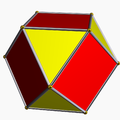Colored faces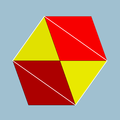3.4.3.4
(Vertex figure)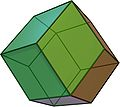Rhombic dodecahedron
(dual polyhedron)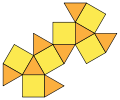Net

In geometry, a cuboctahedron is a polyhedron with eight triangular faces and six square faces. A cuboctahedron has 12 identical vertices, with two triangles and two squares meeting at each, and 24 identical edges, each separating a triangle from a square. As such it is a quasiregular polyhedron, i.e. an Archimedean solid, being vertex-transitive and edge-transitive.

Its dual polyhedron is the rhombic dodecahedron.

## Area and volume

The area A and the volume V of the cuboctahedron of edge length a are:$A = (6+2\sqrt{3})a^2 \approx 9.4641016a^2$$V = \frac{5}{3} \sqrt{2}a^3 \approx 2.3570226a^3.$

## Cartesian coordinates

The Cartesian coordinates for the vertices of a cuboctahedron (of edge length √2) centered at the origin are:

(±1,±1,0)
(±1,0,±1)
(0,±1,±1)

An alternate set of coordinates can be made in 4-space, as 12 permutations of:

(0,1,1,2)

This construction exists as one of 16 orthant facets of the cantellated 16-cell.

### Root vectors

The cuboctahedron's 12 vertices can represent the root vectors of the simple Lie group A3. With the addition of 6 vertices of the octahedron, these vertices represent the 18 root vectors simple Lie group B3.

## Geometric relations

A cuboctahedron can be obtained by taking an appropriate cross section of a four-dimensional 16-cell.

A cuboctahedron has octahedral symmetry. Its first stellation is the compound of a cube and its dual octahedron, with the vertices of the cuboctahedron located at the midpoints of the edges of either.

The cuboctahedron is a rectified cube and also a rectified octahedron.

It is also a cantellated tetrahedron. With this construction it is given the Wythoff symbol: 3 3 | 2.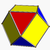A skew cantellation of the tetrahedron produces a solid with faces parallel to those of the cuboctahedron, namely eight triangles of two sizes, and six rectangles. While its edges are unequal, this solid remains vertex-uniform: the solid has the full tetrahedral symmetry group and its vertices are equivalent under that group.

The edges of a cuboctahedron form four regular hexagons. If the cuboctahedron is cut in the plane of one of these hexagons, each half is a triangular cupola, one of the Johnson solids; the cuboctahedron itself thus can also be called a triangular gyrobicupola, the simplest of a series (other than the gyrobifastigium or "digonal gyrobicupola"). If the halves are put back together with a twist, so that triangles meet triangles and squares meet squares, the result is another Johnson solid, the triangular orthobicupola.

Both triangular bicupolae are important in sphere packing. The distance from the solid's centre to its vertices is equal to its edge length. Each central sphere can have up to twelve neighbors, and in a face-centered cubic lattice these take the positions of a cuboctahedron's vertices. In a hexagonal close-packed lattice they correspond to the corners of the triangular orthobicupola. In both cases the central sphere takes the position of the solid's centre.

Cuboctahedra appear as cells in three of the convex uniform honeycombs and in nine of the convex uniform polychora.

The volume of the cuboctahedron is 5/6 of that of the enclosing cube and 5/8 of that of the enclosing octahedron.

## Related polyhedra

The cuboctahedron shares its edges and vertex arrangement with two nonconvex uniform polyhedra: the cubohemioctahedron (having the square faces in common) and the octahemioctahedron (having the triangular faces in common). It also serves as a cantellated Tetrahedron, as being a rectified Tetratetrahedron.

Name tetrahedron rectified tetrahedron
(octahedron)
truncated tetrahedron cantellated tetrahedron
(cuboctahedron)
omnitruncated tetrahedron
(truncated octahedron)
Snub tetrahedron
(icosahedron)
Schläfli {3,3} t1{3,3} t0,1{3,3} t0,2{3,3} t0,1,2{3,3} s{3,3}
Coxeter-DynkinGraph
(A3)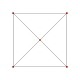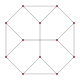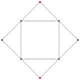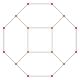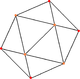Graph
(A2)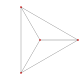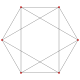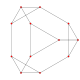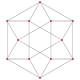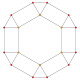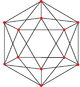Solid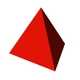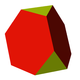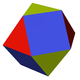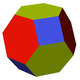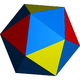Tiling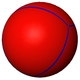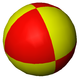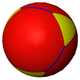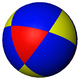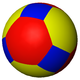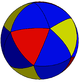The cuboctahedron 2-covers the tetrahemihexahedron, which accordingly has the same abstract vertex figure (2 triangles and two squares: 3.4.3.4) and half the vertices, edges, and faces. (The actual vertex figure of the tetrahemihexahedron is 3.4.3/2.4, with the a/2 factor due to the cross.)

## Related polytopes

The cuboctahedron can be decomposed into a regular octahedron and eight irregular but equal octahedra in the shape of the convex hull of a cube with two opposite vertices removed. This decomposition of the cuboctahedron corresponds with the cell-first parallel projection of the 24-cell into 3 dimensions. Under this projection, the cuboctahedron forms the projection envelope, which can be decomposed into 6 square faces, a regular octahedron, and 8 irregular octahedra. These elements correspond with the images of 6 of the octahedral cells in the 24-cell, the nearest and farthest cells from the 4D viewpoint, and the remaining 8 pairs of cells, respectively.

## Cultural occurrences

• The "Geo Twister" fidget toy  is a flexible cuboctahedron.

Wikimedia Foundation. 2010.

### Look at other dictionaries:

• cuboctahedron — noun A polyhedron made up of 8 triangular and 6 square faces; it has twelve identical vertices …   Wiktionary

• cuboctahedron — cub·octahedron …   English syllables

• cuboctahedron — …   Useful english dictionary

• Truncated cuboctahedron — (Click here for rotating model) Type Archimedean solid Uniform polyhedron Elements F = 26, E = 72, V = 48 (χ = 2) Faces by sides …   Wikipedia

• Cubitruncated cuboctahedron — Type Uniform star polyhedron Elements F = 20, E = 72 V = 48 (χ = −4) Faces by sides 8{6}+6{8}+6{8/3} Wythoff …   Wikipedia

• Great truncated cuboctahedron — In geometry, the great truncated cuboctahedron is a nonconvex uniform polyhedron, indexed as U20. Cartesian coordinates Cartesian coordinates for the vertices of a great truncated cuboctahedron centered at the origin are all permutations of: (±1 …   Wikipedia

• Кубооктаэдр — …   Википедия

• Runcinated tesseract — Tesseract …   Wikipedia

• Convex uniform honeycombs in hyperbolic space — The {5,3,4} honeycomb in 3D hyperbolic space, viewed in perspective In geometry, a convex uniform honeycomb is a tessellation of convex uniform polyhedron cells. In 3 dimensional hyperbolic space there are nine Coxeter group f …   Wikipedia

• Uniform star polyhedron — A display of uniform polyhedra at the Science Museum in London …   Wikipedia